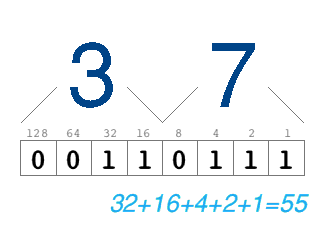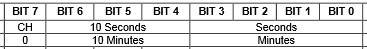# Converting between decimal and binary-coded-decimal (BCD)

When tinkering with Arduinos, you’re going to come across quite a number of components that provide data out – or require data in – Binary Coded Decimal (BCD for short).

If you’re familiar with converting between binary, decimal and hexadecimal then this is fairly straightforward … but if you’re just beginning to tinker with microcontrollers then you may find yourself at a loss. I hope this article will make things a bit clearer.

BCD is where a value isn’t stored in RAM in a simple binary format, but instead in a sort-of half-binary-but-half-decimal way. What is actually happening is that each digit of the number in its decimal form is being stored in a fixed number of bits … usually four or eight.

For example, if you needed to store the decimal number 37 in BCD, with four-bits reserved for each column, then it would be encoded like this:So, if you were to read this byte from RAM (or from another component) and were expecting to get the decimal value of 37, you would actually get … 55. What you need to do is read the byte, then convert it back from BCD to decimal, before using it. Not hard, just a bit confusing.

## Why is this necessary?

It used to be very common on much older computer systems with primitive instruction sets, but not so much anymore. Occasionally you might see it used where mathematics requiring unlimited precision are more important than fitting numbers into fixed-width data formats (such as in financial and scientific applications). But where I see it most frequently is in digital electronics; often involving clocks, or other types of numeric displays. For example: most old pocket-calculators store numbers in BCD, because it makes the display circuitry so much simpler.

Let’s look at how to convert to and from BCD.

## Converting from BCD to Decimal

Lets say you read a byte from a component which the datasheet says is in BCD, with four bits for each digit. The datasheet might not have explicitly said “BCD” – it might have just declared the “tens” separately instead:An excerpt from the DS1307 realtime-clock datasheet, describing data format for minutes and seconds. Though it doesn’t explicitly say “BCD”, it shows that tens-of-units are stored in separate bits (4-6) to the units (0-3).

To convert this into an ordinary decimal value, you separate the digits with some simple binary masking, multiply the tens value by 10, then add them together:

```byte bcd_value = read_from_somewhere();      // lets say bcd_value = 55, or 0x37
byte tens = bcd_value >> 4;                  // tens = 3 (by shifting down the number four places)
byte units = bcd_value & 0x0F;               // units = 7 (the 0x0F filters-out the high digit)
byte final_value = (tens * 10) + units;      // final_value = 37```

A useful thing to point out here is to note that the hexadecimal representation of the BCD value matches the final value. For example: 37 in decimal becomes 0x37 in BCD! If you’re ever using constants in your code which are BCD values, then it’s highly recommended that you use them in their hexadecimal form, as it’ll make it clearer what your code is doing.

## Converting from Decimal to BCD

To do this, we must separate the tens from the units, shift the tens up four places, then binary-OR them together:

```byte orig_value = 35;                 // original value we want to encode in BCD
byte tens = orig_value / 10;          // tens = 3 (by integer division)
byte units = orig_value % 10;         // units = 5 (by modulus)
byte bcd_value = (tens << 4) | units; // bcd_value = 53, or 0x35
(by shifting the tens up four
places and binary-OR'ing it
with the units)
```

## Example code for the Arduino

These two functions are the kind of thing you’ll write once, and then copy-and-paste them into any new project that needs them forever afterwards. I present them here to conclude:

```// turns a standard byte into a BCD equivalent
byte DecToBcd(byte val)
{
return( ((val/10) << 4) | (val%10) );
}

// turns a BCD-encoded byte back into a standard one
byte BcdToDec(byte bcd)
{
return( ((bcd >> 4) * 10) + (bcd&0xF) );
}```